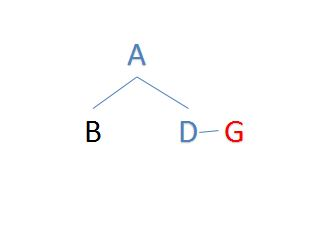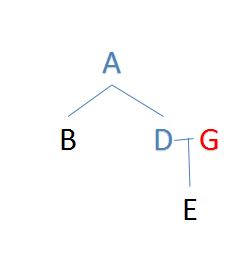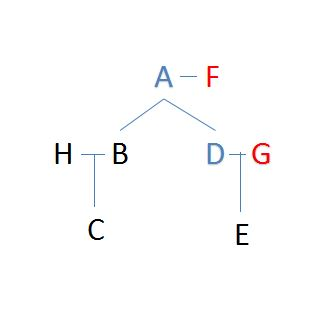0
2166

# Blood Relation Questions For IBPS RRB PO

Download Top-20 IBPS RRB PO Blood Relation Questions PDF. Blood Relation questions based on asked questions in previous year exam papers very important for the IBPS RRB PO (Officer Scale-I, II & III) exam.

Question 1: Introducing Sarita, Meena said, “She is the only daughter of my father’s only daughter”. How is Meena related to Sarita ?

a) Niece

b) Cousin

c) Aunt

e) None of these

Instructions

Study the following information and answer the given questions.
S is the father of R. R is the only son of U. U is the daughter of J. J has only two children. K is the son of J.

Question 2: How is R related to J ?

a) Grandfather

b) Son

c) Nephew

d) Grandson

e) Son-in-law

Instructions

Study the following information and answer the questions. K is the wife of V. V is the brother of J. L is the only daughter of J. D is the father of M and L. S is the only daughter of M.

Question 3: How is D related to V ?

a) Nephew

b) Brother

c) Brother-in-­law

d) Son

e) Father-in-law

Question 4: If S is married to G, how is G related to M ?

a) Daughter

b) Son

c) Nephew

d) Niece

e) Son-­in-­law

Question 5: How is K related to L ?

a) Aunt

b) Niece

c) Mother­in­law

d) Mother

e) Sister

Question 6: X’s mother is the mother-in-law of the father of Z. Z is the brother of Y while X is the father of M. How is X related to Z ?

a) Paternal uncle

b) Maternal uncle

c) Cousin

d) Grandfather

e) Brother-in-law

Question 7: If A is the brother of B, C is the sister of A, D is the brother of E, E is the daughter of B, F is the father of C. who is the uncle of D ?

a) A

b) C

c) B

d) None of these

e) Can’t be determined

Question 8: A said to B that B’s mother was the mother-in-law of A’s mother. How is A’s mother related to B’s mother ?

a) Daughter-in-law

b) Mother-in-law

c) Sister

d) Aunt

e) None of these

Instructions

<p “=””>Study the following information and answer the questions given. B is the mother of T. T is the sister of J. J is married to L. L is the only son of K. K is the daughter of P.

Question 9: How is T related to L ?

a) Cousin

b) Sister

c) Sister-in-law

d) Cannot be determined

e) Niece

Question 10: How is B related to L ?

a) Mother

b) Mother-in-law

c) Sister-in-law

d) Grandmother –

e) Cannot be determined

Question 11: If K is the mother of X, then how is X related to P ?

a) Son

b) Grandson

c) Daughter

d) Granddaughter

e) Cannot be determined

Question 12: B is the father of Q. B has only two children. Q is the brother of R. R is the daughter of P. A is the granddaughter of P. S is the father of A. How is S related to Q?

a) Son

b) Son-in-law

c) Brother

d) Brother-in-law

e) None of these

Question 13: L is the daughter of K. L is married to D. B is the son of D. F is married to B. How is D related to F?

a) Father-in-law

b) Mother-in-law

c) Cannot be determined

d) Father

e) Mother

Instructions

A family consists of 8 members – A, B, C, D, E, F, G, and H. There are 3 married couples in the family. A and B do not belong to the same generation. B and D are siblings. G is married to D. A has a granddaughter and a grandson. A is B’s father and G is E’s mother. Except A, none of the persons have more than 1 child. E and H belong to different generations. A is married to F.

Question 14: If B is the mother of C, then how is H related to F?

a) Son in law

b) father in law

c) Son

d) daughter

e) None of these

Question 15: Which of the following statements is definitely false?

a) E is the grand son of A.

b) C is the grandson of A.

c) H is the father of C.

d) B is the father of C.

e) G is the mother of C.

Question 16: What is the difference between the number of male and female members in the family?

a) 1

b) 2

c) 0

d) 3

e) Cannot be determined.

Instructions

Study the following information carefully and answer the questions given below :
S has two daughters R and A. G is married to R. G is father of N. L is son of A.

Question 17: How L is related to S ?

a) Nephew

b) Brother

c) Grandson

d) Great grandson

e) None of these

Question 18: Which of the following statement is/are true on the basis of information given above ?

a) G is son-in-law of S

b) R is wife of G

c) A is mother of L

d) L is cousin of N

e) All are true

Meena’s father’s only daughter = Meena herself

Now Sarita is the daughter of Meena

=> Meena is Sarita’s mother.

=> Ans – (E)

S is the father of R. R is the only son of U, => U is the wife of S.

U is the daughter of J. J has only two children. K is the son of J, => K is the brother of U.

R is the son of J’s daughter, => R is the grandson of J.

=> Ans – (D)

K is the wife of V. V is the brother of J.

L is the only daughter of J. D is the father of M and L, => D is the husband of J and M is the brother of L.

S is the only daughter of M.

D is the husband of V’s sister, => D is the brother-in-law of V.

=> Ans – (C)

K is the wife of V. V is the brother of J.

L is the only daughter of J. D is the father of M and L, => D is the husband of J and M is the brother of L.

S is the only daughter of M.

If S is married to G, then G is the son-in-law of M.

=> Ans – (E)

K is the wife of V. V is the brother of J.

L is the only daughter of J. D is the father of M and L, => D is the husband of J and M is the brother of L.

S is the only daughter of M.

K is the aunt of L.

=> Ans – (A)

X is the father of M, => X is male.

X’s mother is the mother-in-law of the father of Z, => The father of Z is married to X’s sister.

Thus, X is the brother of Z’s mother, hence X is the maternal uncle of Z.

=> Ans – (B)

A is the brother of B and C is the sister of A, => A (male) , B and C (female) are siblings.

D is the brother of E and E is the daughter of B, => D (male) and E (female) are the children of B.

F is the father of C, => F is the father of A, B and C.

Thus, A is the uncle of D.

=> Ans – (A)

B’s mother was the mother-in-law of A’s mother, => B is the father of A.

Hence, A’s mother is the daughter-in-law of B’s mother.

=> Ans – (A)

B is the mother of T. T is the sister of J.

J is married to L and L is the only son of K, => J is female and daughter of B.

Also, K is the daughter of P.

T is the sister of L’s wife, thus T is the sister-in-law of L.

=> Ans – (C)

B is the mother of T. T is the sister of J.

J is married to L and L is the only son of K, => J is female and daughter of B.

Also, K is the daughter of P.

B is the mother of L’s wife, thus B is the mother-in-law of L.

=> Ans – (B)

B is the mother of T. T is the sister of J.

J is married to L and L is the only son of K, => J is female and daughter of B.

Also, K is the daughter of P.

If K is the mother of X, then X must be a girl, since L is the only son of K, thus X is granddaughter of P.

=> Ans – (D)

B is the father of Q and Q is the brother of R. Also, R is the daughter of P

=> B is husband of P and their children are Q(male) and R(female).

A is the granddaughter of P and S is the father of A

=> S is husband of R and their daughter is A.

S is married to Q’s sister, => S is brother-in-law of Q.

=> Ans – (D)

L is the daughter of K. L is married to D, => D is the husband of L.

B is the son of D and F is married to B, => B is the son of D and L and F is the wife of B.

Thus, D is the father-in-law of F.

=> Ans – (A)

A has a granddaughter and grandson. A is B’s father. Hence, A must be the grandfather. B and D are siblings. G is D’s wife. Hence, D is a male member.(Male members are represented by blue colour and female members are represented by red colour. Persons whose gender cannot be determined are represented by black colour.)

G is E’s mother. E can either be the grandson or the granddaughter of A.A is married to F. Hence, F is a female member. H and E do not belong to the same generation. Hence, H must be the spouse of B. The final arrangement is as follows.If B is the mother of C, then H is B’s husband. Hence, H is the son-in-law of F.

A has a granddaughter and grandson. A is B’s father. Hence, A must be the grandfather. B and D are siblings. G is D’s wife. Hence, D is a male member.(Male members are represented by blue colour and female members are represented by red colour. Persons whose gender cannot be determined are represented by black colour.)

G is E’s mother. E can either be the grandson or the granddaughter of A.A is married to F. Hence, F is a female member. H and E do not belong to the same generation. Hence, H must be the spouse of B. The final arrangement is as follows.As we can see from the diagram, G is the mother of E. Hence, G cannot be the mother of C (No one has more than 1 child except A).

A has a granddaughter and grandson. A is B’s father. Hence, A must be the grandfather. B and D are siblings. G is D’s wife. Hence, D is a male member.(Male members are represented by blue colour and female members are represented by red colour. Persons whose gender cannot be determined are represented by black colour.)

G is E’s mother. E can either be the grandson or the granddaughter of A.A is married to F. Hence, F is a female member. H and E do not belong to the same generation. Hence, H must be the spouse of B. The final arrangement is as follows.A is a male member and F is a female member.
B and H is a couple. Hence, one among them must be a male and the other must be a female member.
D is a male member and G is a female member.
It is given that A has a grandson and grand daughter. Hence, one among C and E must be a male member and the other must be a female member.

As we can see, there is an equal number of male and female members in the family. Hence, 0 is the right answer.

S has two daughters R and A. G is married to R, => G is the husband of R.

G is father of N and L is son of A.

L is the grandson of S.

=> Ans – (C)